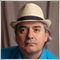# Strange behavior in calculating the bid price in the strategy tester91

I'm testing an EA in the strategy tester.

To get a graphical representation of it, I also added an indicator, a simple indicator that warns me when the bid price breaks the lower or upper limits of a donchian channel. In real time, the indicator works perfectly, but in the strategy tester the signals are completely wrong.

Going to investigate I realized that the calculation of the bid price is wrong. In practice, to check if the bid price is above the superior limit of the Donchian, I use an IF. The problem is that the bid price is not of the graph that is forming in the strategy tester, but it is the real bid of the instrument in real time! I tried to get the bid in different ways: directly with Bid, or with = MarketInfo (Symbol (), MODE_BID), but it does not change anything .. I do not understand this strange behavior ..4980

pieroim:

I'm testing an EA in the strategy tester.

To get a graphical representation of it, I also added an indicator, a simple indicator that warns me when the bid price breaks the lower or upper limits of a donchian channel. In real time, the indicator works perfectly, but in the strategy tester the signals are completely wrong.

Going to investigate I realized that the calculation of the bid price is wrong. In practice, to check if the bid price is above the superior limit of the Donchian, I use an IF. The problem is that the bid price is not of the graph that is forming in the strategy tester, but it is the real bid of the instrument in real time! I tried to get the bid in different ways: directly with Bid, or with = MarketInfo (Symbol (), MODE_BID), but it does not change anything .. I do not understand this strange behavior ..

You will have to show your code so that we can help you! It is impossible to see what you are doing wrong without the code reference.

Also remember that you should be using the the previous bar's High/Low values of the Donchian channel (Bar shift = 1, not 0), otherwise it will just be constantly changing.91

```//+------------------------------------------------------------------+
//| Custom indicator iteration function                              |
//+------------------------------------------------------------------+
int OnCalculate(const int rates_total,
const int prev_calculated,
const datetime &time[],
const double &open[],
const double &high[],
const double &low[],
const double &close[],
const long &tick_volume[],
const long &volume[],
{
//---

int limit = rates_total - prev_calculated;
if(prev_calculated > 0) limit++;

for(int i=0; i < limit; i++)
{
upper[i]=iHigh(Symbol(),Period(),iHighest(Symbol(),Period(),MODE_HIGH,candele,i));
lower[i]=iLow(Symbol(),Period(),iLowest(Symbol(),Period(),MODE_LOW,candele,i));
middle[i] = (upper[i]+lower[i])/2;
}

OutputDebugStringW("Bid : " + Bid);
if (pausa==true)//routine x uso in testing pausa
{
datetime data_corrente = iTime(Symbol(), Period(), 0);
if (data_corrente>(lastTimeC+minuti_attesa))//filtro x impedire segnale su tutti i tick della candela di rottura + le candelle di attesa definite in extern
{
double bid = Bid;
OutputDebugStringW(bid);
double upper_persistenza, lower_persistenza;
bool upper_pers, lower_pers;
int upper_cont = 1;
int lower_cont = 1;
for (int y=1; y<min_lung_donc; y++)//in realtá valuta 7 candele se impostato a 8, esclusa l'ultima, la 0
{
upper_persistenza = iHigh(Symbol(),Period(),iHighest(Symbol(),Period(),MODE_HIGH,candele,y));
lower_persistenza = iLow(Symbol(),Period(),iLowest(Symbol(),Period(),MODE_LOW,candele,y));
if (upper_persistenza==upper)
{
upper_cont +=1;
}
if (lower_persistenza==lower)
{
lower_cont +=1;
}
}
if (upper_cont==min_lung_donc) upper_pers=true;
if (lower_cont==min_lung_donc) lower_pers=true;
if (bid>upper && upper_pers==true)//rottura rialzista
{
lastTimeC = iTime(Symbol(),Period(), 0);
PauseTest();
OutputDebugStringW(bid + "  UP  " + upper);
}
if (bid<lower && lower_pers==true)//rottura ribassista
{
lastTimeC = iTime(Symbol(),Period(), 0);
PauseTest();
OutputDebugStringW(bid + "  DOWN  " + lower);
}
}
}

datetime data = iTime(NULL, Period(), candela_testo);
if (data > lastTime)
{
lastTime = data;
ObjectMove(0,nome_,0,data,lower[candela_testo]);
}

//--- return value of prev_calculated for next call
return(rates_total);
}
//+------------------------------------------------------------------+
void OnDeinit(const int reason)
{
ObjectDelete(0,nome_);
}```91

#import "kernel32.dll"
void OutputDebugStringW(string sMsg);
#import